# How do I input a linear equation using the built-in template ax + b = cx + d?

Select the "ax + b = cx + d" format in the Settings window.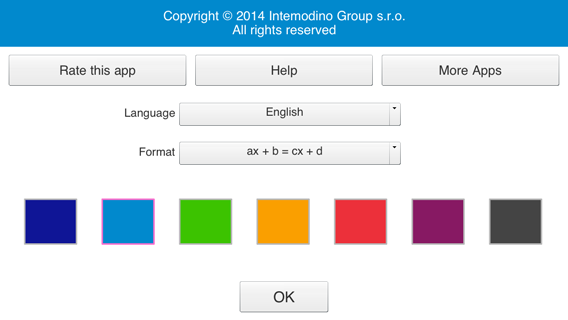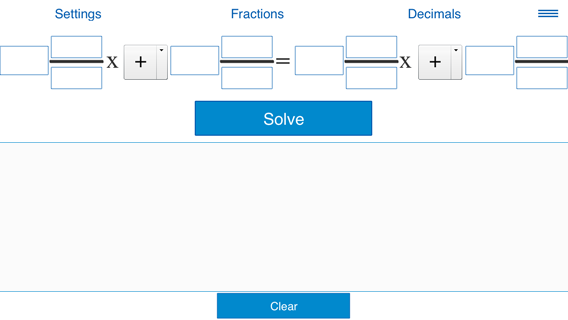Note: This window is available in the landscape orientation only.

Enter the values for a, b, c and d and click "Solve".

a, b, c and d could be whole numbers, fractions or decimals.

This format supports two modes: "Fractions" (coefficients could be whole numbers and fractions) and "Decimals" (coefficients could be whole numbers or decimals). Pressing "Fractions" and "Decimals" switches between the two modes.

The following examples show how to input equations.

Example 1. Solving the equation
2x - 1.5 = 3x + 2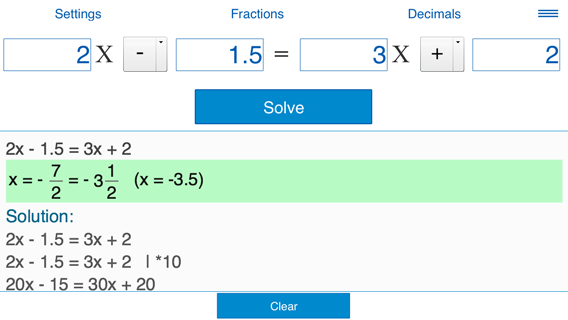Example 2. Solving the equation
3.5 = 10x - 1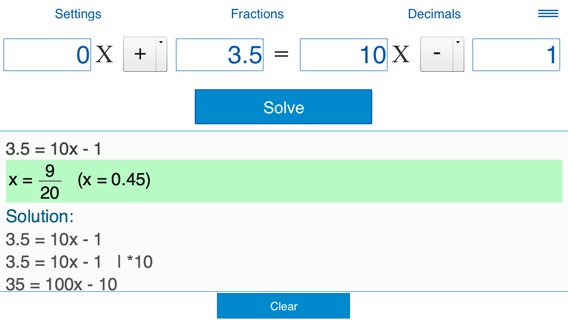Example 3. Solving the equation
4x + 5 = 3.5x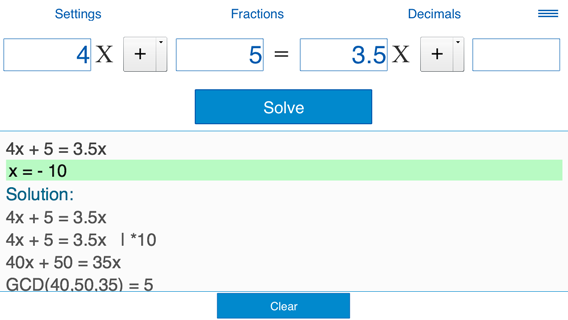Example 4. Solving the equation
1/2
x - 1
1/4
= -x - 5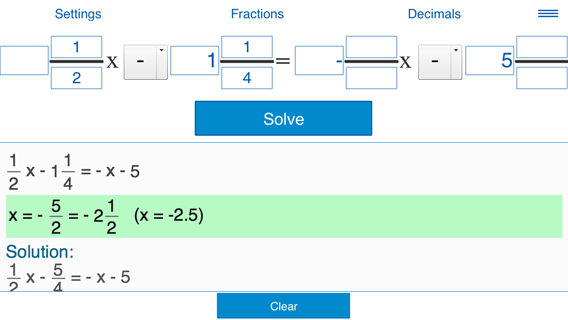Monday, December 4, 2023

Contact